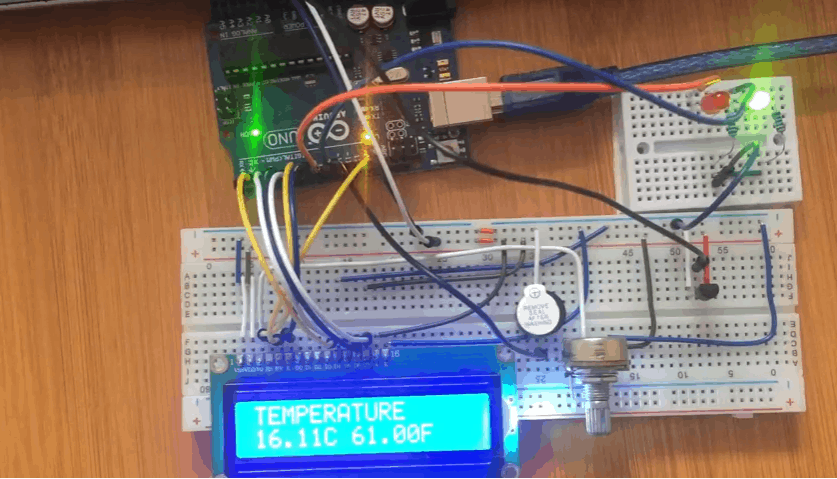# LM35 Temperature sensor Arduino digital Thermometer.In this project we have made an Arduino based digital thermometer to display the current ambient temperature and temperature changes on a LCD unit in real time. It can be deployed in houses, offices, industries etc. to measure the temperature. This project is based on Arduino which communicates here with LM35 temperature sensor, 16×2 LCD display unit and leds.

This sensor is used for sensing environment temperature which gives 1 degree temperature on every 10mV change at its output pin. You can easily check it with voltmeter by connecting Vcc at pin 1 and Ground at pin 3 and output voltage at pin 2 of LM35 sensor. For an example if the output voltage of LM35 sensor is 250m volt, that means the temperature is around 25 degree Celsius.

Arduino reads output voltage of temperature sensor by using Analog pin A0 and performs the calculation to convert this Analog value to a digital value of current temperature. After calculations arduino sends these calculations or temperature to 16×2 LCD unit by using appropriate commands of LCD.

## Code for LM35 Temperaturesensor with Arduino and LCD

```#include <LiquidCrystal.h>
LiquidCrystal lcd(12,11,5,4,3,2);

const int inPin = A0; //sensor
#define buzzer 10
#define redled 8
#define greenled 9
int sound = 250;

void setup()
{
pinMode(redled, OUTPUT);
pinMode(greenled, OUTPUT);
pinMode(buzzer, OUTPUT);
lcd.begin(16,2);
lcd.setCursor(0,0);
lcd.print("THERMOMETER");
}

void loop()
{
float millivolts = (value / 1024.0) * 5000;
float t = millivolts / 10;  // temperature in degrees celcius
lcd.setCursor(0,1);
lcd.print(t);
lcd.write(0xdf); lcd.print("C ");
lcd.print((t * 9)/5 + 32); // converting celcius to fahrenheit
lcd.write(0xdf); lcd.print("F");
delay(1000); //Updating Temperature reading after every 2 seconds

if (t<=27) //leds and buzzer setup process
{
digitalWrite(redled, LOW);
digitalWrite(greenled, HIGH);
noTone(buzzer);
}
else if (t>=29)
{
digitalWrite(redled, HIGH);
digitalWrite(greenled, LOW);
tone(buzzer, sound);
}

}
```

When this code is uploaded to the Arduino, the LCD display will show the temperature in degrees Celcius and Fahrenheit. When the temperaute goes above a required value, the red led lights and the buzzer sounds.